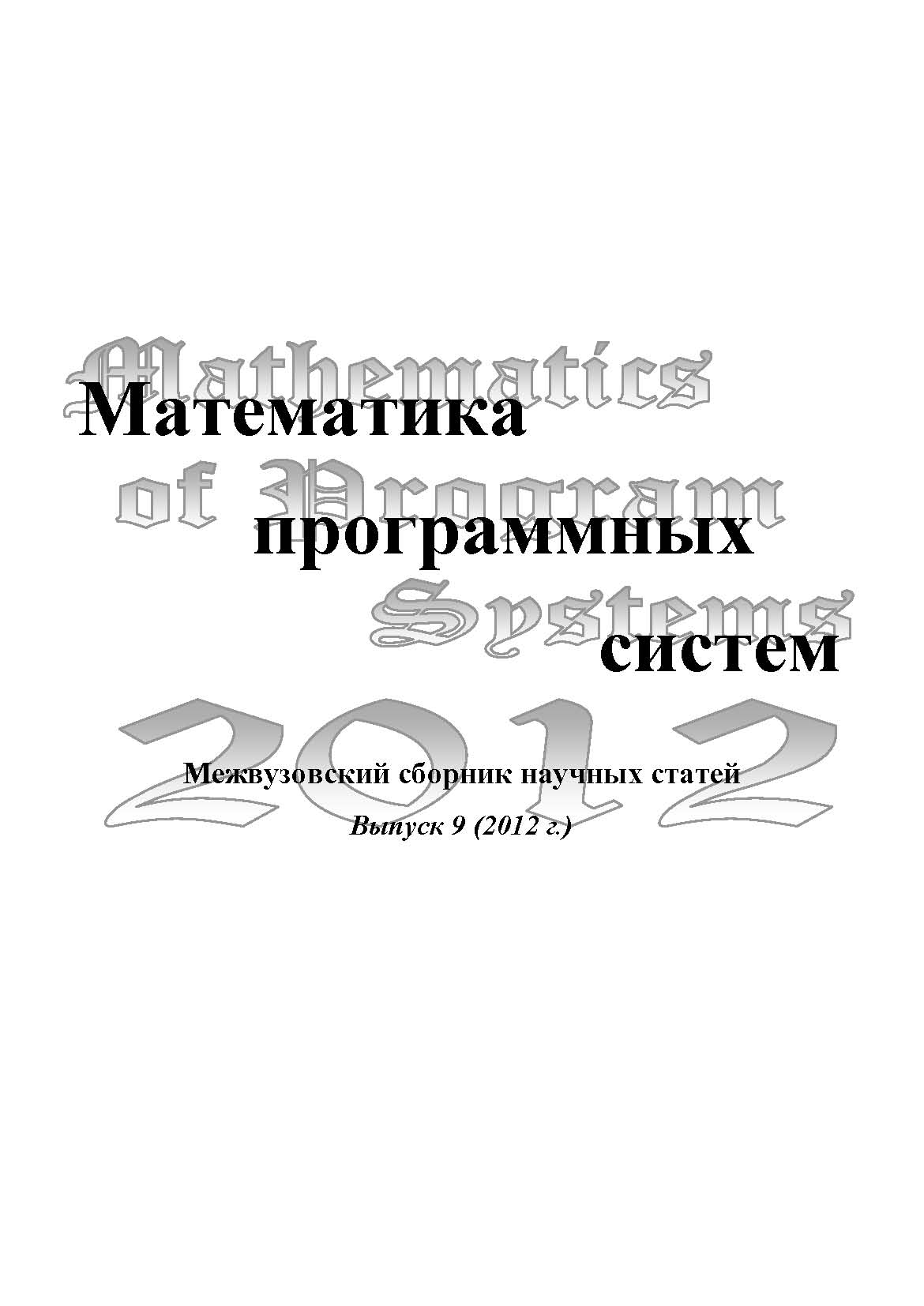• A
• A
• A
• ABC
• ABC
• ABC
• А
• А
• А
• А
• А
Regular version of the site

## Решение систем трансцендентных уравнений с помощью генетических алгоритмов

С. 170-178.
Морозенко В. В., Сюткин А. А.

In this research we analyzed the problem of solving transcendental equations' systems. More detailed is analyzed the approach of solution based on genetic algorithms because it is less examined than the one based on numerical methods. The research will be useful for different kinds of physical and mathematical calculations containing transcendental equations of high complexity. The research is available for students and graduates who are familiar with the basics of numerical methods, mathematical analysis, discrete mathematics and combinatorial algorithms.

### In bookEdited by: А. И. Миков, Л. Н. Лядова Вып. 9. Пермь: Пермский государственный национальный исследовательский университет, 2012.